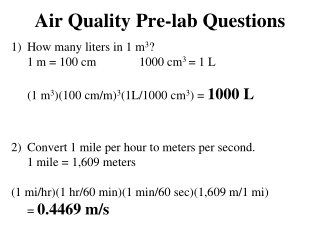DownloadDownload PresentationAir Quality Pre-lab Questions

Air Quality Pre-lab Questions

Download PresentationAir Quality Pre-lab Questions

- - - - - - - - - - - - - - - - - - - - - - - - - - - E N D - - - - - - - - - - - - - - - - - - - - - - - - - - -
Presentation Transcript

1. Air Quality Pre-lab Questions • How many liters in 1 m3? 1 m = 100 cm 1000 cm3 = 1 L (1 m3)(100 cm/m)3(1L/1000 cm3) = 1000 L • Convert 1 mile per hour to meters per second. 1 mile = 1,609 meters (1 mi/hr)(1 hr/60 min)(1 min/60 sec)(1,609 m/1 mi) = 0.4469 m/s

2. 3) What is the PSI for air with 0.267 ppm SO2? PSI = (upper PSI value – lower PSI value) X (pollutant concentration at upper PSI – pollutant concentration at lower PSI) (observed pollutant concentration – pollutant concentration at lower PSI) + PSI at lower pollutant concentration = (200 – 100) X (0.267 ppm – 0.14 ppm) + 100 (0.30 ppm – 0.14 ppm) = (625)(0.127 ppm) + 100 = 179.38 PSI Descriptor = Unhealthful

3. 4) What % of smoke is CO by volume? 1 vol·percent = 10,000 ppm (3100 ppm)(1%/10,000 ppm) = 0.31 % by volume

4. #5 Conversions – do these first Minutes to seconds 15 min = 900 sec Diameter (cm)to radius(m) 1.5cm/2 = 0.75cm = 0.0075m Celsius to Kelvin 20  C + 273 = 293 K % volume to Liters 3100 ppm = 0.31% = 0.0031 L

5. 5) ***You are finding the total volume of the air produced*** • V = (flow rate)(cross section of air stream)(time) = (0.52 m/s)(π)(r)2 (900 sec) = (0.52 m/s)(π)(0.0075m)2 (900 sec) = (0.52 m/s)(0.000177 m2)(900 sec) = (0.083 m3)(1000 L/ m3) = 83 L of air expelled Next, find volume of CO emitted (volume of the pollutant) V = (83 L)(vol % CO) = (83 L)(0.0031) = 0.26 L CO

6. 5b) moles CO emitted PV = nRT R = 0.08206 L*atm /mol*K (1 atm)(0.26 L CO) = n(0.08206 L*atm /mol*K)(293 K) 0.26 = n(24.04) n = 0.0108 moles CO 5c) mass of CO Mass = (n)(molecular weight) = (0.0108 mol CO)(28 g/mol) = 0.30 g CO